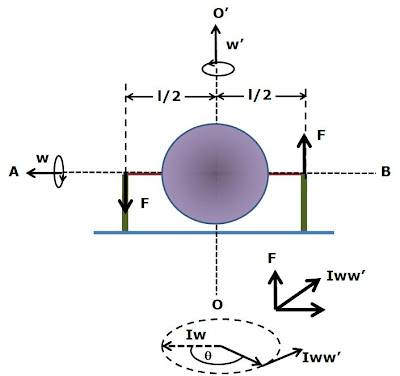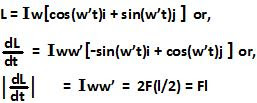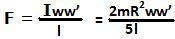## Sunday, March 29, 2009

### Irodov Problem 1.286Let the moment of inertia of the sphere be I. The sphere undergoes two spinning motions, i) its rotation about AB with an angular velocity w (the angular momentum vector Iw is directed along the axis AB as shown) ii) its rotation about OO' with an angular velocity w' (the vector oriented along OO').

As the axis AB rotates a result, the angular momentum vector Iw rotates with it in the plane at a rate w'. Since the angular momentum vector rotates, this can only occur because of torque acting at the bearings. The torque is directed along the tangent of the circle spanned by the tip of angular momentum vector. Since torque is given by F x r (r is directed along AB), F must be directed along the z-axis. Hence, we have,Hence, we have,(see the list of moments of inertia).